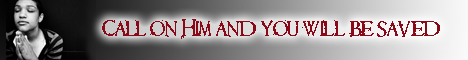Note:  Do not rely on this information. It is very old.

# Algebra

Algebra, in its extended sense, the science of numbers treated symbolically. The symbols are used simply for abbreviation. Hence we may regard algebra as a universal arithmetic worked in a shorthand system. The signs used for certain operations are to be regarded as purely arbitrary and conventional. It follows that the laws of arithmetic must apply to algebra. But in arithmetic the only unit employed is +1, whereas in algebra it is found necessary to introduce others. Thus Descartes introduced the negative unit -1, which is defined as the quantity that when added to the positive unit gives us zero, and which when multiplied by itself gives us +1. Since his time the use of another unit Ö - 1 has been found necessary. This is defined as the quantity that gives us - 1 when multiplied by itself. In the science of quaternions (q.v.) other units are introduced, with, however, perfectly defined characteristics. Besides these units it is necessary to have a code of invariable laws that shall govern all operations performed with them. Thus in arithmetic we have 2 x 3 x 4 = 3 x 4 x 2, that is, any product of any multiples of the unit 1 is the same, whatever order we take to perform the multiplication. So in algebra we have abc = bca = cab. The three chief laws are (a) the commutative law. Additions and subtractions, or multiplications and divisions, may be made in any order, (b) The distributive law. The multiplication of a sum of terms is the sum of the multiplications of each term; so also with division of a sum of terms, (c) The law of indices. The product of two powers of a number is that number raised to the sum of the powers.